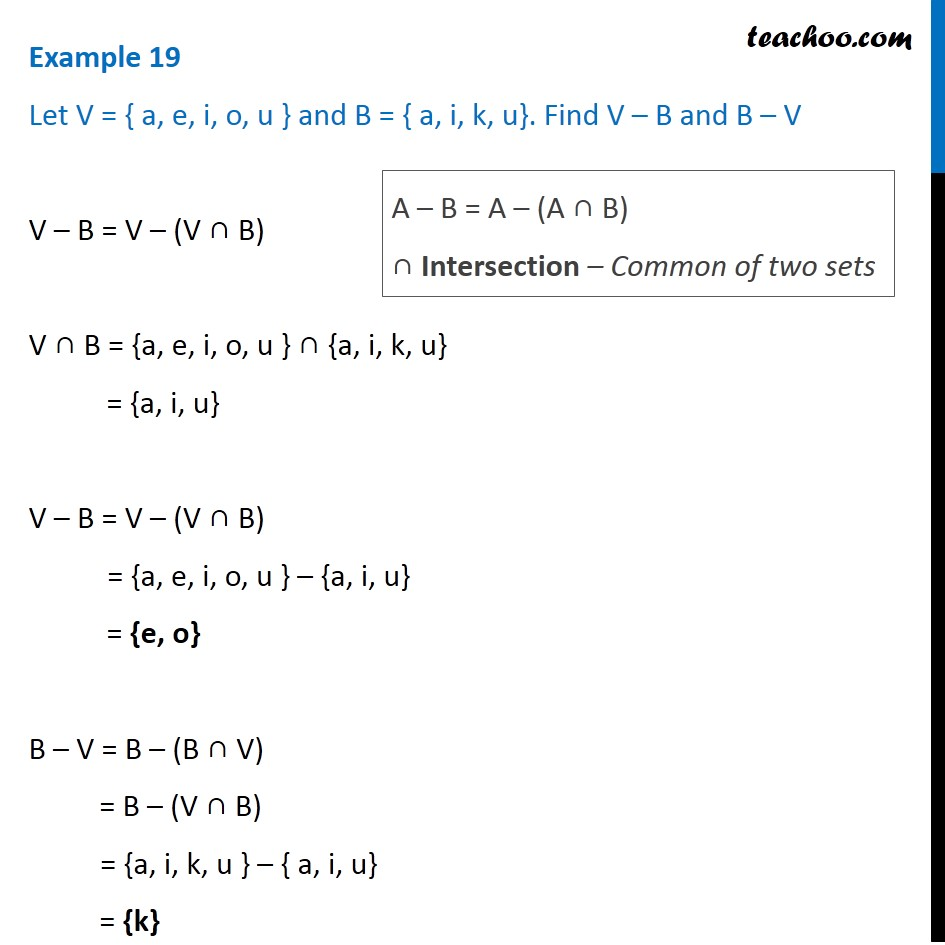Examples

Chapter 1 Class 11 Sets
Serial order wiseLearn in your speed, with individual attention - Teachoo Maths 1-on-1 Class

### Transcript

Example 19 Let V = { a, e, i, o, u } and B = { a, i, k, u}. Find V – B and B – V V – B = V – (V ∩ B) V ∩ B = {a, e, i, o, u } ∩ {a, i, k, u} = {a, i, u} V – B = V – (V ∩ B) = {a, e, i, o, u } – {a, i, u} = {e, o} B – V = B – (B ∩ V) = B – (V ∩ B) = {a, i, k, u } – { a, i, u} = {k} A – B = A – (A ∩ B) ∩ Intersection – Common of two sets